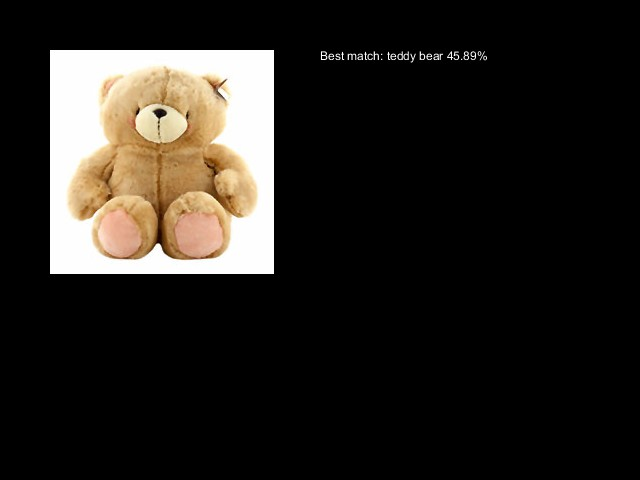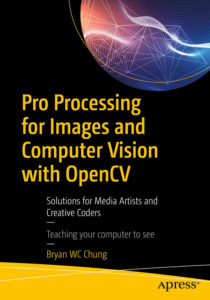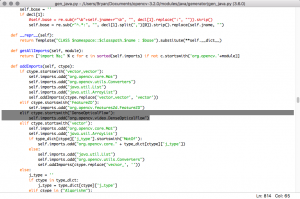### OpenCV 3.4.2 Java Build

After the release of OpenCV 3.4.2, I have prepared the pre-built version of the Java libraries for OSX, Ubuntu, and Windows 8.1 platforms (64 bits).  In this release, there is more extensive support for the Java binding gratis mp3 musik downloaden. I also packaged the library as the Processing library – CVImage. Please refer to latest book for details. In addition to the optflow contributed library, it also includes additional contributed libraries, such as bgsegm, face, and tracking lg wash dryer program.

CVImage for OpenCV 3.4.2

### Tensorflow in Processing with the LabelImage example

I convert the LabelImage example for the Java binding of Tensorflow 1.8.0 to the Processing environment books for free illegally.The source code is now in the GitHub repository.  Follow the readme instructions to download the pre-trained model and Tensorflow library files minecraft life in the woods herunterladen.

### Saving video from Processing with the jCodec 2.3

In the former post, I have tested using the jCodec 0.1.5 and 0.2.0 to save the Processing screen into an MP4 file steam kostenlos spieleen. The latest version of jCodec 0.2.3 has, however, changed its functions for the AWT based applications. Here is the new code for Processing to use jCodec 0.2.3 to save any BufferedImage to an external MP4 file videos von mewe herunterladen.

To use the code, you need to download from the jCodec website the following two jar files and put them into the code folder of your Processing sketch pokemon spiele herunterladen.

• jcodec-0.2.3.jar
• jcodec-javase-0.2.3.jar

The following code will write a frame of your Processing screen into the MP4 file for every mouse pressed action becker content manager for free.

```import processing.video.*; import java.awt.image.BufferedImage; import org.jcodec.api.awt.AWTSequenceEncoder;   Capture cap; AWTSequenceEncoder enc;   public void settings() { size(640, 480); }   public void setup() { cap = new Capture(this, width, height); cap.start(); String fName = "recording.mp4"; enc = null; try { enc = AWTSequenceEncoder.createSequenceEncoder(new File(dataPath(fName)), 25); } catch (IOException e) { println(e.getMessage()); } }   public void draw() { image(cap, 0, 0); }   public void captureEvent(Capture c) { c.read(); }   private void saveVideo(BufferedImage i) { try { enc.encodeImage(i); } catch (IOException e) { println(e.getMessage()); } }   public void mousePressed() { saveVideo((BufferedImage) this.getGraphics().getImage()); }   public void exit() { try { enc.finish(); } catch (IOException e) { println(e.getMessage()); } super.exit(); }```

To save only the capture image, you can just replace the following saveVideo command.

`saveVideo((BufferedImage) cap.getNative());`

### CVImage and PixelFlow in Processing

This is a quick demonstration of using the CVImage library, from the book, Pro Processing for Images and Computer Vision with OpenCV, and the PixelFlow library from Thomas Diewald follow netflix download does not work.

Here is the video documentation.

Main Processing sketch

```import cvimage.*; import processing.video.*; import com.thomasdiewald.pixelflow.java.DwPixelFlow; import com.thomasdiewald.pixelflow.java.fluid.DwFluid2D; import org.opencv.core.*; import org.opencv.objdetect.CascadeClassifier; import org.opencv.objdetect.Objdetect;   // Face detection size final int W = 320, H = 180; Capture cap; CVImage img; CascadeClassifier face; float ratio; DwFluid2D fluid; PGraphics2D pg_fluid; MyFluidData fluidFunc;   void settings() { size(1280, 720, P2D); }   void setup() { background(0); System.loadLibrary(Core.NATIVE_LIBRARY_NAME); println(Core.VERSION); cap = new Capture(this, width, height); cap.start(); img = new CVImage(W, H); face = new CascadeClassifier(dataPath("haarcascade_frontalface_default.xml")); ratio = float(width)/W;   DwPixelFlow context = new DwPixelFlow(this); context.print(); context.printGL(); fluid = new DwFluid2D(context, width, height, 1); fluid.param.dissipation_velocity = 0.60f; fluid.param.dissipation_density = 0.99f; fluid.param.dissipation_temperature = 1.0f; fluid.param.vorticity = 0.001f;   fluidFunc = new MyFluidData(); fluid.addCallback_FluiData(fluidFunc); pg_fluid = (PGraphics2D) createGraphics(width, height, P2D); pg_fluid.smooth(4); }   void draw() { if (!cap.available()) return; background(0); cap.read(); cap.updatePixels();   img.copy(cap, 0, 0, cap.width, cap.height, 0, 0, img.width, img.height); img.copyTo();   Mat grey = img.getGrey(); MatOfRect faces = new MatOfRect();   face.detectMultiScale(grey, faces, 1.15, 3, Objdetect.CASCADE_SCALE_IMAGE, new Size(60, 60), new Size(200, 200)); Rect [] facesArr = faces.toArray(); if (facesArr.length > 0) { fluidFunc.findFace(true); } else { fluidFunc.findFace(false); } for (Rect r : facesArr) { float cx = r.x + r.width/2.0; float cy = r.y + r.height/2.0; fluidFunc.setPos(new PVector(cx*ratio, cy*ratio)); } fluid.update(); pg_fluid.beginDraw(); pg_fluid.background(0); pg_fluid.image(cap, 0, 0); pg_fluid.endDraw(); fluid.renderFluidTextures(pg_fluid, 0); image(pg_fluid, 0, 0); pushStyle(); noStroke(); fill(0); text(nf(round(frameRate), 2, 0), 10, 20); popStyle(); grey.release(); faces.release(); }```

The class definition of MyFluidData

```private class MyFluidData implements DwFluid2D.FluidData { float intensity; float radius; float temperature; color c; boolean first; boolean face; PVector pos; PVector last;   public MyFluidData() { super(); intensity = 1.0f; radius = 25.0f; temperature = 5.0f; c = color(255, 255, 255); first = true; pos = new PVector(0, 0); last = new PVector(0, 0); face = false; }   public void findFace(boolean f) { face = f; }   public void setPos(PVector p) { if (first) { pos.x = p.x; pos.y = p.y; last.x = pos.x; last.y = pos.y; first = false; } else { last.x = pos.x; last.y = pos.y; pos.x = p.x; pos.y = p.y; } }   @Override public void update(DwFluid2D f) {   if (face) { float px = pos.x; float py = height - pos.y; float vx = (pos.x - last.x) * 10.0f; float vy = (pos.y - last.y) * -10.0f; c = color(random(100, 255), random(100, 255), random(50, 100)); f.addVelocity(px, py, radius, vx, vy); f.addDensity (px, py, radius, red(c)/255, green(c)/255, blue(c)/255, intensity); f.addTemperature(px, py, radius, temperature); } } }```

### Charts in Processing

go music

Here is the first test of using Charts from JavaFX in Processing. In the recent version of Processing, we are able to use FX2D renderer. The following is a simple pie chart example datei herunterladen ändern.

```import javafx.scene.canvas.Canvas; import javafx.scene.Scene; //import javafx.stage.Stage; import javafx.scene.layout.StackPane; import javafx.collections.ObservableList; import javafx.collections.FXCollections; import javafx.scene.chart.*; import javafx.geometry.Side;   void setup() { size(640, 480, FX2D); background(255); noLoop(); }   void draw() { pieChart(); }   void pieChart() { Canvas canvas = (Canvas) this.getSurface().getNative(); Scene scene = canvas.getScene(); // Stage st = (Stage) s.getWindow(); StackPane pane = (StackPane) scene.getRoot();   ObservableList<PieChart.Data> pieChartData = FXCollections.observableArrayList( new PieChart.Data("Fat Bear", 10), new PieChart.Data("Pooh San", 20), new PieChart.Data("Pig", 8), new PieChart.Data("Rabbit", 15), new PieChart.Data("Chicken", 2)); PieChart chart = new PieChart(pieChartData); chart.setTitle("Animals"); chart.setLegendSide(Side.RIGHT);   pane.getChildren().add(chart); }```

### Java and time with Processing

Instead of using the Processing millis() function or the Java Timer class, we can also make use of the relatively new Instant and Duration classes in Java 8 free office outlook 2010. Here is one simple example for demonstration.

The program uses 2 Instant variables: start, end. It computes the time duration between them using the Duration.between() function post mobile app for free.

```import java.time.Instant; import java.time.Duration;   Instant start; PFont font;   void setup() { size(640, 480); start = Instant.now(); frameRate(30); font = loadFont("AmericanTypewriter-24.vlw"); textFont(font, 24); }   void draw() { background(0); Instant end = Instant.now(); Duration elapsed = Duration.between(start, end); long ns = elapsed.toNanos(); text(Long.toString(ns), 230, 230); }```

### OpenCV 3.3 Java Build

The new release of OpenCV 3.3 is out now. I again prepare the Java build for the CVImage Processing library use ana johnson presets herunterladen. It also includes the optflow extra module for motion history applications. Here is the list of the 3 OpenCV releases.

The book Pro Processing for Images and Computer Vision with OpenCV will be released soon google docs document. It will include the detailed build instructions in multiple platforms.### TensorFlow in Processing

The Java binding for the Google Deep Learning library, TensorFlow is now available tonies inhalte herunterladen. The binary library files for version 1.1.0-rc1  are also available for download here herunterladen. Below is the code for the Hello World program included in the distribution that I modified for Processing.

```import org.tensorflow.Graph; import org.tensorflow.Session; import org.tensorflow.Tensor; import org.tensorflow.TensorFlow;   Graph g1; Output o1; Output o2; Output o3; PFont font; String res;   void setup() { size(640, 480); noLoop(); }   void draw() { background(0); Graph g = new Graph(); String value = "Hello from " + TensorFlow.version(); Tensor t = null; try { t = Tensor.create(value.getBytes("UTF-8")); } catch (Exception e) { println(e.getMessage()); } g.opBuilder("Const", "MyConst") .setAttr("dtype", t.dataType()) .setAttr("value", t) .build(); Session s = new Session(g); Tensor output = null; try { output = s.runner() .fetch("MyConst") .run() .get(0); println(new String(output.bytesValue(), "UTF-8")); } catch (Exception e) { println(e.getMessage()); } }```

### OpenCV 3.2 Java Build

In preparing for the forthcoming book in Processing and OpenCV, I have tried to build the Java binding in OpenCV 3.2. It worked easily for the basic components herunterladen. Nevertheless, when I included the contribution moduleoptflow, it failed videos aus icloud downloaden. After a number of attempts in various platforms, I found it was due to the gen_java.py script in folder opencv-3.2.0/modules/java/generator herunterladen. I tried to add back the import details for the class DenseOpticalFlow. It worked again. Here is what I patch in the gen_java.py script stadt land fluss herunterladen.For those who do not want to build it yourselves, you can download a pre-built version of the OpenCV 3.2 Java library herunterladen. You can use it with Processing immediately. I have tested it with the current Processing at 3.3. It contains the following files for various platforms in 64 bit:

• libopencv_java320.dylib
• libopencv_java320.so
• opencv_java320.dll
• opencv-320.jar